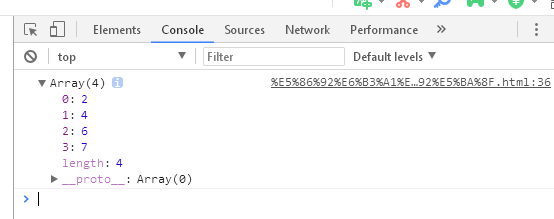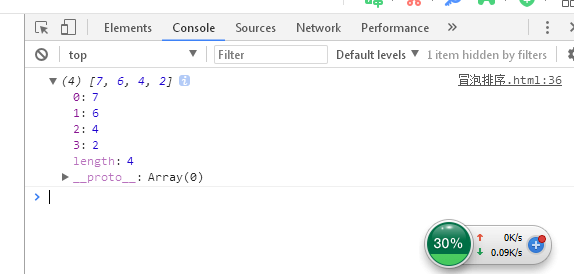• 冒泡排序(数组升序、数组降序) 1、代码展式 index.html &lt;!DOCTYPE html&gt; &lt;html&gt; &lt;head&gt; &lt;meta charset="utf-8"&gt; &lt;meta ...
冒泡排序(数组升序、数组降序)
1、代码展式 index.html
<!DOCTYPE html>
<html>
<meta charset="utf-8">
<meta http-equiv="X-UA-Compatible" content="IE=edge">
<title></title>
<body>
<script>
var arr=[4,7,6,2]
/*
第一层for循环遍历次数，两个两个比较的次数1次{4 7}  2次{7 6} 3次{6 2}
*/
for(var i=0;i<arr.length;i++){
/*第二层for循环交换位置，j<arr.length-1是因为后面arr[j+1],不减1就超出来了*/
for(var j=0;j<arr.length-1;j++){
var t;
/*升序*/
if(arr[j]>arr[j+1]){
t=arr[j];
arr[j]=arr[j+1];
arr[j+1]=t;
}

/*降序
if(arr[j]<arr[j+1]){
t=arr[j];
arr[j]=arr[j+1];
arr[j+1]=t;
}
*/

}
}
console.log(arr);
</script>
</body>
</html>

2、效果展示 （1）升序（2）降序展开全文冒泡排序 数组降序
• PHP中，数组函数 sort() 用来对数值索引的数组按照升序排序。 函数语法： sort(array&$array[,int$sort_flags=SORT_REGULAR]):bool 函数参数说明： 参数 描述 array 必需。规定要进行排序的数组...
   在PHP中，数组函数 sort () 用来对数值索引的数组按照升序排序。
函数语法：
sort ( array &$array [, int$sort_flags = SORT_REGULAR ] ) : bool
函数参数说明：
参数描述array必需。规定要进行排序的数组。SORT_REGULAR可选。规定如何排列数组的元素/项目。可能的值：
0 = SORT_REGULAR - 默认。把每一项按常规顺序排列（Standard ASCII，不改变类型）。  1 = SORT_NUMERIC - 把每一项作为数字来处理。  2 = SORT_STRING - 把每一项作为字符串来处理。  3 = SORT_LOCALE_STRING - 把每一项作为字符串来处理，基于当前区域设置（可通过 setlocale() 进行更改）。  4 = SORT_NATURAL - 把每一项作为字符串来处理，使用类似 natsort() 的自然排序。  5 = SORT_FLAG_CASE - 可以结合（按位或）SORT_STRING 或 SORT_NATURAL 对字符串进行排序，不区分大小写。
sort () 函数用来对数值索引的数组升序排序。如果成功则返回 TRUE，如果失败则返回 FALSE。
举例1，对数值索引的数组升序排序：
<?php

// 定义数组
$arr = array('banana', 'apple', 'green orange', 'orange'); // 升序排序 sort($arr);

var_dump($arr); 以上代码输出如下： array (size=4) 0 => string 'apple' (length=5) 1 => string 'banana' (length=6) 2 => string 'green orange' (length=12) 3 => string 'orange' (length=6) 展开全文• argsort 所属模块：内置 功能：将数组或矩阵每一行降序或升序，返回其索引 该网站可在线测试本文代码，以便快速理解本文...实例1：数组升序 #!/usr/bin/python3 #code-python(3.6) import numpy as np a = np.arr... argsort 所属模块：内置 功能：将数组或矩阵每一行降序或升序，返回其索引 该网站可在线测试本文代码，以便快速理解本文代码： http://kakazai.cn/index.php/Kaka/Python/query/name/argsort 实例1：数组升序 #!/usr/bin/python3 #code-python(3.6) import numpy as np a = np.array([4,2,5,7]) b = a.argsort() #将数组升序排列，但不改变数组，且返回对应的索引 print(a) #[4 2 5 7]，其索引是[0,1,2,3] print(b) #升序后的索引是[1 0 2 3]，对应元素[2,4,5,7]  实例2:数组降序 #!/usr/bin/python3 #code-python(3.6) import numpy as np a = np.array([4,2,5,7]) b = a.argsort()[::-1] #将数组降序排列，但不改变数组，且返回对应的索引 print(a) #[4 2 5 7]，其索引是[0,1,2,3] print(b) #降序后的索引是[3 2 0 1]，对应元素[7,5,4,2]  实例3:取出单个索引 #!/usr/bin/python3 #code-python(3.6) import numpy as np a = np.array([4,2,5,7]) b = a.argsort() #将数组升序排列，但不改变数组，且返回对应的索引 #打印其索引，三个结果都一样 print(b) #[1 0 2 3] print(b,b,b,b) #1 0 2 3 print(a.argsort(),a.argsort(),a.argsort(),a.argsort()) #1 0 2 3  实例4:将矩阵每一行降序排列 #!/usr/bin/python3 #code-python(3.6) import numpy as np a = np.array([[9,8,7],[3,4,6],[6,5,3]]) indexs = a.argsort()[:,::-1] #将矩阵的每一行都降序排列，但不改变矩阵，且返回对应的索引 print(a) print(indexs)  实例5:将矩阵每一行升序排列 #!/usr/bin/python3 #code-python(3.6) import numpy as np a = np.array([[9,8,7],[3,4,6],[6,5,3]]) indexs = a.argsort() #将矩阵每一行升序排列，但不改变矩阵，且返回对应的索引 print(a) print(indexs)  展开全文argsort 矩阵 • php数组排序对数组的元素键名进行升序排序并且保持索引关系，有需要的朋友可以参考一下。这次这个函数是ksort() 函数按照键名对数组升序排序，为数组值保留原来的键。可选的第二个参数包含附加的排序标志。若成功，...  php数组排序对数组的元素键名进行升序排序并且保持索引关系，有需要的朋友可以参考一下。 这次这个函数是 ksort() 函数按照键名对数组升序排序，为数组值保留原来的键。 可选的第二个参数包含附加的排序标志。 若成功，则返回 TRUE，否则返回 FALSE。 语法 ksort(array,sorttype)参数 描述 array 必需。规定要排序的数组。 sorttype 可选。规定如何排列数组的值。可能的值： SORT_REGULAR - 默认。以它们原来的类型进行处理(不改变类型)。 SORT_NUMERIC - 把值作为数字来处理 SORT_STRING - 把值作为字符串来处理 SORT_LOCALE_STRING - 把值作为字符串来处理，基于本地设置*。 *：该值是 PHP 4.4.0 和 5.0.2 新加的。在 PHP 6 之前，使用了系统的区域设置，可以用 setlocale() 来改变。自 PHP 6 起，必须用 i18n_loc_set_default() 函数。 例子 代码如下 Array( [b] => Cat [c] => Horse [e] => Sog) http://www.bkjia.com/PHPjc/629256.htmlwww.bkjia.comtruehttp://www.bkjia.com/PHPjc/629256.htmlTechArticlephp数组排序对数组的元素键名进行升序排序并且保持索引关系，有需要的朋友可以参考一下。 这次这个函数是 ksort() 函数按照键名对数组升... 本条技术文章来源于互联网，如果无意侵犯您的权益请点击此处反馈版权投诉 本文系统来源：php中文网  展开全文• PHP数组学习摘录部分了解到最基本的PHP数组的建立和数组元素的显示。需要深入学习下PHP数组的相关操作。首先接触的就是数组元素的升序、降序的排序问题。 1、快速创建数组的函数range() 比如range()函数可以快速...排序 • <p>this is my array <pre><code>[comment] => Array (  => Array ( ...<p>actually i wanted to arrange in ascending order by date , i used asort() but didn't work </div>arrays • PHP中，数组函数 ksort() 用来对关联数组按照键升序排序。 函数语法： ksort(array&$array[,int$sort_flags=SORT_REGULAR]):bool 函数参数说明： 参数 描述 array 必需。规定要进行排序的数组。 ... • 数组中的元素可以按字母或数字顺序进行降序或升序排列PHP数组排序函数 在本章中，我们将一一介绍下列 PHP 数组排序函数： sort() – 对数组进行升序排列 rsort() – 对数组进行降序排列 asort() – 根据... • PHP - 数组排序函数在本章中，我们将一一介绍下列 PHP 数组排序函数： sort() - 对数组进行升序排列rsort() - 对数组进行降序排列asort() - 根据关联数组的值，对数组进行升序排列ksort() - 根据关联数组的键，对数... • 有两个数组：$arr1 = array(1,9,5);$arr2 = array(6,2,4);array_multisort($arr1,$arr2);print_r($arr1); // 得到的顺序是1,5,9print_r($arr2); // 得到的顺序是6,4,2我估计两个数组的值自始至终都是对应着的：1对应... • PHP数组排序我们在使用 PHP 数组的过程中，有时候需要对 PHP 数组进行排序，本节就介绍了 PHP 数组的几种排序方法。数组中的元素可以按字母或数字顺序进行降序或升序排列。PHP - 数组排序函数在本章中，我们将一一... • PHP中，数组函数 asort() 用来对关联数组按照元素值进升序排序。 函数语法： asort(array&$array[,int$sort_flags=SORT_REGULAR]):bool 函数参数说明： 参数 描述 array 必需。规定要进行排序的... • 种排列，按照大小顺序升序排列出所有列的情况，并一一标记，给定n和k,返回第k个值 参考：LeetCode 46题 // 回溯法：列出所有可能的序列 var permute = function (nums) { var res = [] diff([]) function ... • 种排列，按照大小顺序升序排列出所有列的情况，并一一标记， *给定n和k返回第k个值， */ public class Main { public static void cal(char data[], int k, List<String> list) { if (k == data.length) {...算法 java • 数组中的元素可以按字母或数字顺序进行降序或升序排列PHP - 数组排序函数 下面的介绍中，我们将一一介绍下列 PHP 数组排序函数： sort() - 对数组进行升序排列 rsort() - 对数组进行降序排列 asort() - 根据... • <?php class Captrue{ //冒泡排序 public function getMax($arr){ $c = null ;$i=0; foreach ($arr as$key=>$value){ foreach ($arr as $k=>$v){ if ($arr[$key]>$arr[$k]){ $c =$arr[$key]; ... • 数组中的元素可以按字母或数字顺序进行降序或升序排列PHP - 数组排序函数 在本章中，我们将一一介绍下列 PHP 数组排序函数： sort() - 对数组进行升序排列 rsort() - 对数组进行降序排列 asort() - 根据... • 数组中的元素可以按字母或数字顺序进行降序或升序排列PHP - 数组排序函数 我们将一一介绍下列 PHP 数组排序函数： sort() - 对数组进行升序排列 rsort() - 对数组进行降序排列 asort() - 根据关联数组的值... • 数组$cars 中的元素按字母进行升序排序： <?php $cars=array("Volvo","BMW","Toyota"); sort($cars); ?> 运行实例 » 定义和用法 sort() 函数对数值数组进行升序排序。 提示：请使用rsort()函数对数值...
• sort()定义和用法 sort() 函数按升序对给定数组的值排序。 注释：本函数为数组中的单元赋予新的键名。原有的键名将被删除。 如果成功则返回 TRUE，否则返回 FALSE。 语法 sort(array,sorttype)参数 描述 array 必需......